## For Math Expressions

Math expressions are much simpler and easier with OptoScript. Some of the operations are built right into the language, such as Add and Multiply.

OptoScript has no limitations on the number of parentheses you can use in math expressions.

Here’s an example of a mathematical expression in OptoScript:

integer1 = (integer2 + 2) * (float1 / (float2 - 2) - 3);

Now compare that to the commands needed in an action block to do the same computation: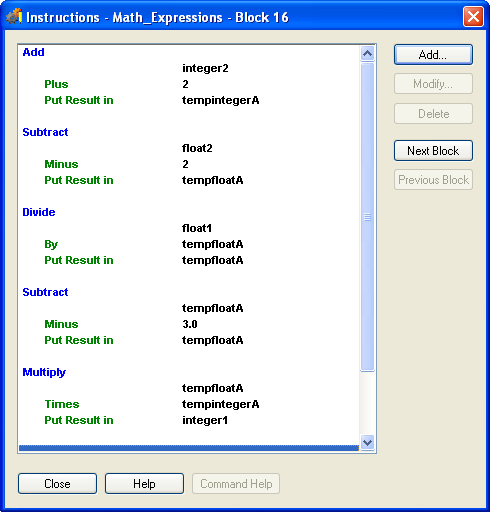As you can see, the OptoScript code is easier to create and to understand.

OptoScript Expressions and Operators

OptoScript includes mathematical expressions as well as comparison, logical, and bitwise operators. Because
expressions and operators are built into the OptoScript language, several standard PAC Control commands
such as Multiply, Bit Shift, and Greater Than or Equal? aren't used.

Using Mathematical Expressions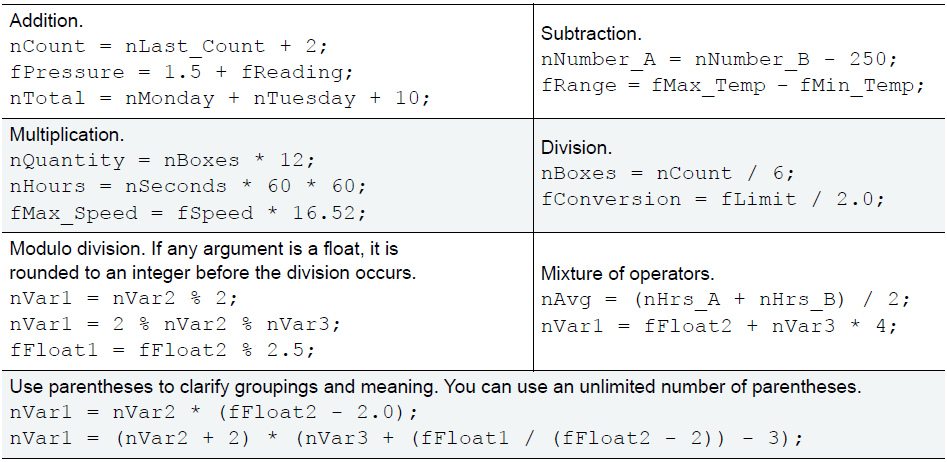Using Comparison Operators

All OptoScript comparison operators return an Integer 32 value of zero (flase) or of non-zero (true). OptoScript supports the following comparison operators for comparing two numeric values: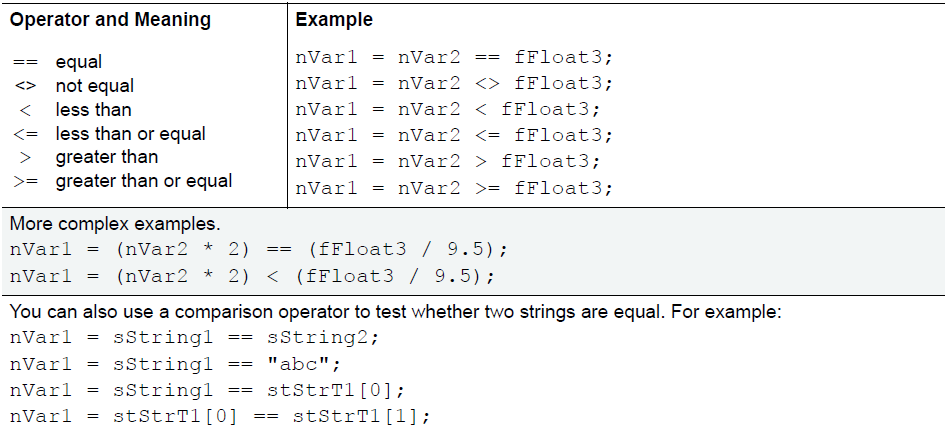Note: Except for the equal (==) operator, the comparison operators can only be used with a numeric expression. They can't be used with a null because it isn't considered a number.

Using Logical Operators

All OptoScript logical operators return an Integer 32 value of zero (false) or of non-zero (true). OptoScript supports the following logical operators for numeric values: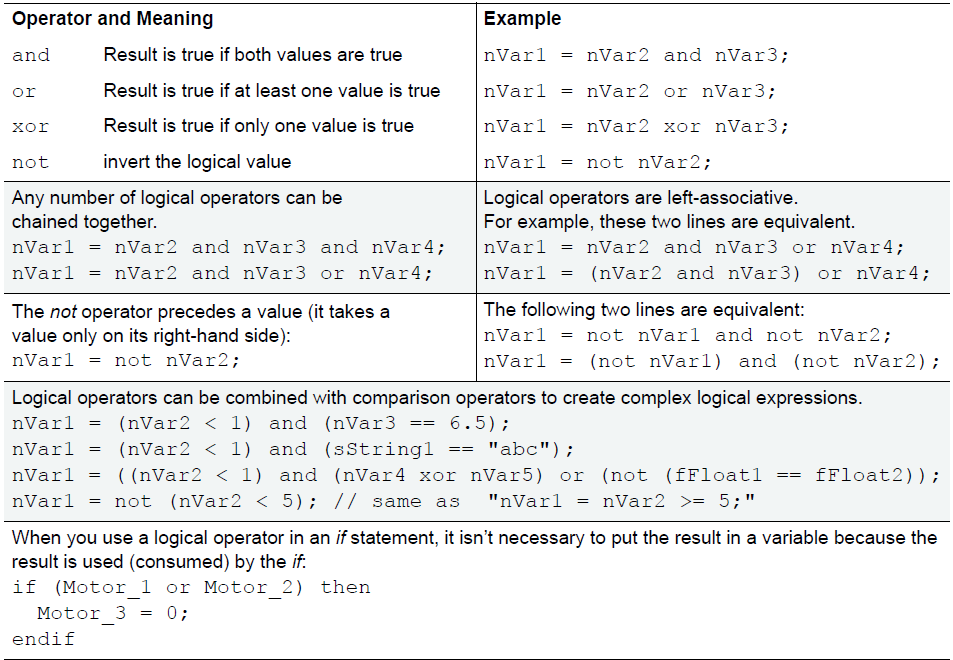Using Bitwise Operators

All OptoScript bitwise operators operate on integer values. OptoScript supports these bitwise operators: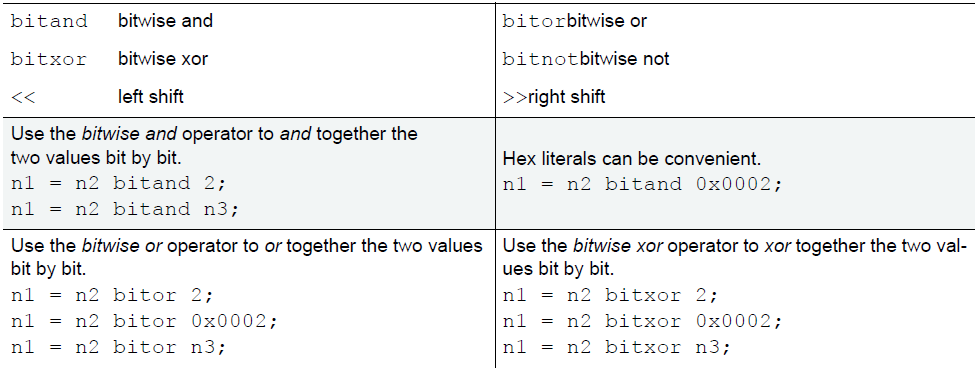For More About OptoScript Math Expressions

A complete description about OptoScript math expressions can be found in the PAC Control User's Guide, Chapter 11: Using OptoScript, OptoScript Expressions and Operators.

#### Summary

Learn about using OptoScript for math expressions. OptoScript includes mathematical expressions as well as comparison, logical, and bitwise operators.